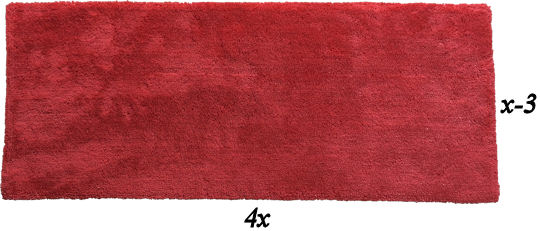# Exam-Style Questions on Quadratic Equations

## Problems on Quadratic Equations adapted from questions set in previous Mathematics exams.

### 1.

IGCSE Extended

(a) Show that the equation $$\frac{3}{x+1}+\frac{3x-9}{2}=1$$ can be simplified to $$3x^2-8x-5=0$$.

(b) Solve the equation $$3x^2-8x-5=0$$ showing all of your working and giving answers to three significant figures.

(c) The total surface area of a cone with radius $$x$$ and slant height $$8x$$ is equal to the area of a circle with radius r. Show that $$r = 3x$$.

[The curved surface area, $$A$$, of a cone with radius $$r$$ and slant height $$l$$ is $$A=\pi rl$$.]

### 2.

IB StudiesA red rug has a width of $$x-3$$ cm and a length of $$4x$$ cm.

(a) Write down an expression for the area, A, in cm2, of the rug.

The area of the rug is 3240 cm2.

(b) Calculate the value of $$x$$.

(c) Hence, write down the value of the length and of the width of the rug in centimetres.

If you would like space on the right of the question to write out the solution try this Thinning Feature. It will collapse the text into the left half of your screen but large diagrams will remain unchanged.

The exam-style questions appearing on this site are based on those set in previous examinations (or sample assessment papers for future examinations) by the major examination boards. The wording, diagrams and figures used in these questions have been changed from the originals so that students can have fresh, relevant problem solving practice even if they have previously worked through the related exam paper.

The solutions to the questions on this website are only available to those who have a Transum Subscription.

Exam-Style Questions Main Page

Search for exam-style questions containing a particular word or phrase:

To search the entire Transum website use the search box in the grey area below.